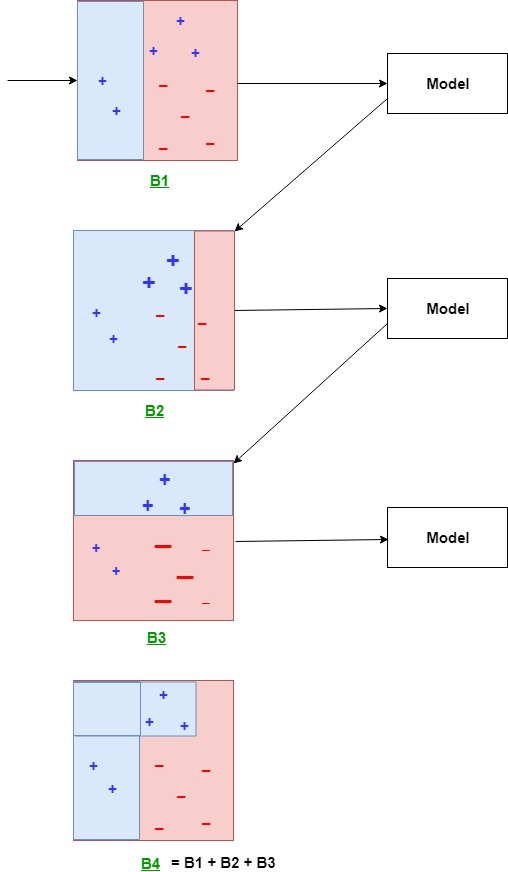Related Articles
Boosting in Machine Learning | Boosting and AdaBoost
• Last Updated : 06 May, 2019

Boosting is an ensemble modeling technique which attempts to build a strong classifier from the number of weak classifiers. It is done building a model by using weak models in series. Firstly, a model is built from the training data. Then the second model is built which tries to correct the errors present in the first model. This procedure is continued and models are added until either the complete training data set is predicted correctly or the maximum number of models are added.

AdaBoost was the first really successful boosting algorithm developed for the purpose of binary classification. AdaBoost is short for Adaptive Boosting and is a very popular boosting technique which combines multiple “weak classifiers” into a single “strong classifier”. It was formulated by Yoav Freund and Robert Schapire. They also won the 2003 Gödel Prize for their work.

Algorithm:

1. Initialise the dataset and assign equal weight to each of the data point.
2. Provide this as input to the model and identify the wrongly classified data points.
3. Increase the weight of the wrongly classified data points.

.

4. if (got required results)
Goto step 5
else
Goto step 2
5. EndExplanation:
Above diagram explains the AdaBoost algorithm in a very simple way. Let’s try to understand it in a step wise process:

• B1 consist of 10 data points which consist of two types namely plus(+) and minus(-) and 5 of which are plus(+) and other 5 are minus(-) and each one has been assigned equal weight initially. The first model tries to classify the data points and generates a vertical separator line but it wrongly classifies 3 plus(+) as minus(-).
• B2 consists of the 10 data points from the previous model in which the 3 wrongly classified plus(+) are weighted more so that the current model tries more to classify these pluses(+) correctly. This model generates a vertical separator line which correctly classifies the previously wrongly classified pluses(+) but in this attempt, it wrongly classifies two minuses(-).
• B3 consists of the 10 data points from the previous model in which the 3 wrongly classified minus(-) are weighted more so that the current model tries more to classify these minuses(-) correctly. This model generates a horizontal separator line which correctly classifies the previously wrongly classified minuses(-).
• B4 combines together B1, B2 and B3 in order to build a strong prediction model which is much better than any individual model used.My Personal Notes arrow_drop_up